GeeksforGeeks App
Open AppBrowser
Continue

# How to Extract Text Only from Alphanumeric String in Excel?

Most often we receive “ID fields” as alphanumeric strings.  We have to extract only text from “ID fields” for further process.

In this article we use two approaches (Excel formula – Substitute function and Excel VBA – User-defined function ) to extract text only from Alphanumeric strings.

## Approach 1:  Using Excel formula

The above-discussed problem statement can be resolved using inbuilt excel formulas as discussed below:

Syntax:

`SUBSTITUTE (text, old_text, new_text, [instance_num])`

Where,

• text: It is the input cell(text).
• old_text: It is the text to find.
• new_text: It is the text to replace.

### Implementation:

Step 1: Open Excel.

Step 2: Type any alphanumeric string in cell “B5” (eg. geeksId345768).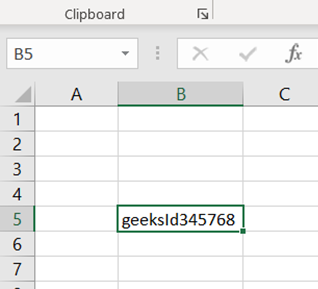Step 3: Write below formula in cell “C5” (we used nested substitute function to replace numbers 0 to 9)

`= SUBSTITUTE(SUBSTITUTE( SUBSTITUTE( SUBSTITUTE( SUBSTITUTE(SUBSTITUTE( SUBSTITUTE( SUBSTITUTE( SUBSTITUTE( SUBSTITUTE(B5,"0",""),"1",""),"2",""), "3",""),"4",""),"5",""), "6",""),"7",""),"8",""),"9","")`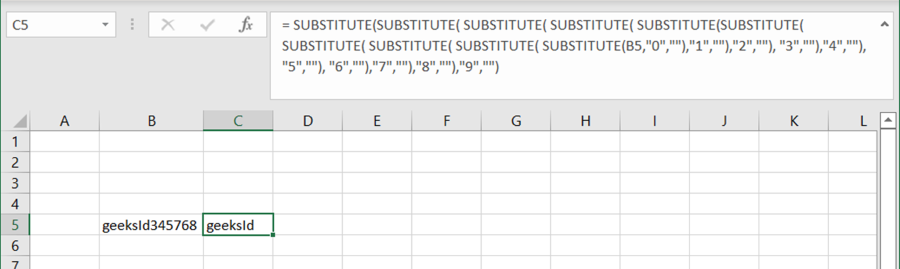## Approach 2: Excel VBA user-defined function

In Excel VBA we can define functions to resolve the problem statement. An implementation is given below for the same.

### Implementation:

Step 1: Open Excel.

Step 2: Type any alphanumeric string in cell “B5” (eg. geeksId345768).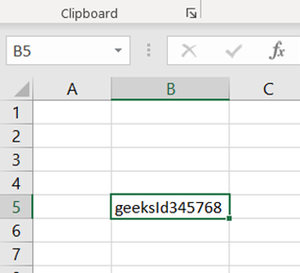Step 3: Write below VBA code in the module:

```Function onlyText(rg As Range) As String

Dim ipVal As String
Dim opValue As String```
• Initialize variable
`ipVal = rg.Value`
• Iterate the input string
`For i = 1 To Len(ipVal)`
•  Check the character is Numeric or not
```   If Not IsNumeric(Mid(ipVal, i, 1)) Then
opValue = opValue & Mid(ipVal, i, 1)
End If
Next```
• Return output
```onlyText = opValue
End Function```

Step 4: Save your excel file as “Excel Macro-Enabled Workbook”  [*.xlsm]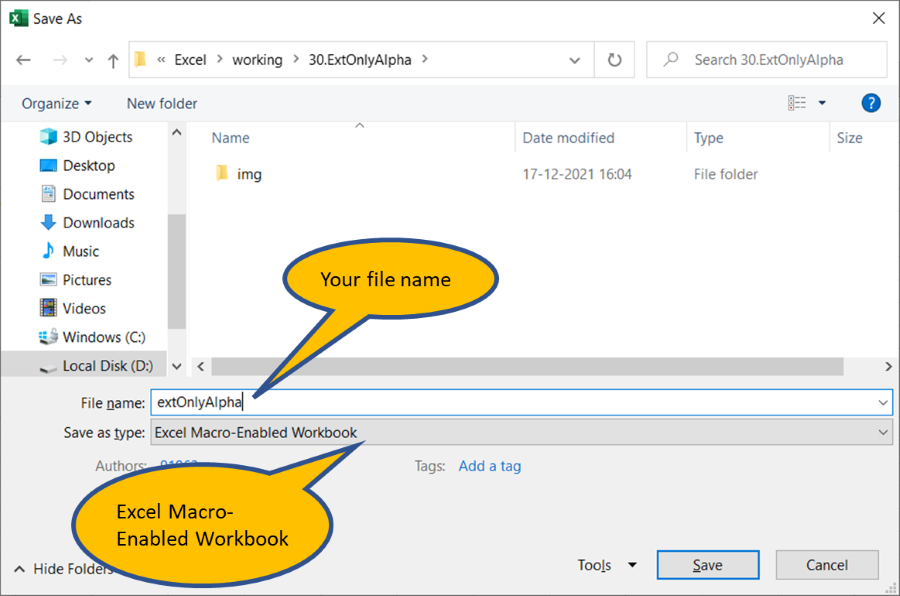Step 5: Type the function name “=onlyText(B5)” in cell “C5”.

Macro remove all numeric character from cell “B5” and return to cell “C5”.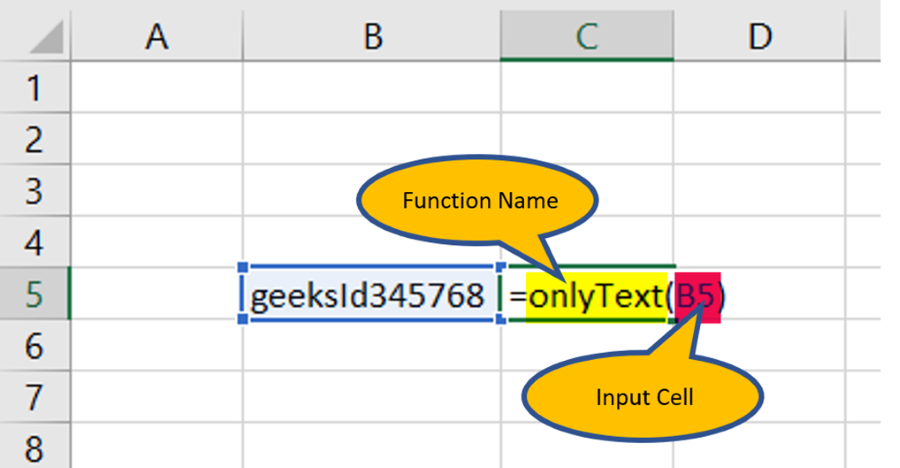My Personal Notes arrow_drop_up